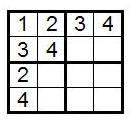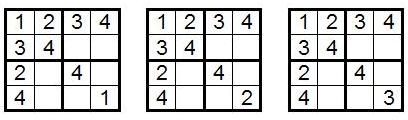### Contents

Home

1. Introduction to Sudoku

2. Solving Strategy

3. Counting Solutions

4. Solution Symmetries

5. The 4×4 Case

6. Some More Interesting Facts

7. References

# The Math Behind Sudoku

## The 4×4 Case

Let us look at the Sudoku of rank 2, or the 4×4 Sudoku grid, in order to get a better understanding of the ideas we have discussed so far.

In order to count the number of 4×4 Sudoku grids, we start - as in the 9×9 case - by filling in the top left block in the standard way. This accounts for a factor of 4! in the number of distinct grids, since there are 4! ways to relabel four digits.

After doing this, 1 and 2 occur in the first two entries of the first row, leaving 3 and 4 to go in the second two entries. For each of the 4! labelings, there are two ways to insert 3 and 4 into two cells, and this contributes to the count of distinct grids. However, for a count of essentially different grids, the order in which we enter 3 and 4 here does not matter, since swapping the third and fourth columns preserves the validity of the grid. So we may assume that 3 goes in the third cell and 4 in the fourth cell of the first row.

Since 1 and 3 occur in the first column of the grid already, 2 and 4 must be placed in the third and fourth cells of that column. Since there are two ways to do this, our count of distinct grids is now up to 4!×2×2. For counting essentially different grids, we note that swapping the third and fourth rows is a symmetry of the grid, so we may assume that 2 goes in the third cell and 4 in the fourth cell of the first column. At this point, we have the following grid:Since there is a 2 in the third row and a 3 in the third column, the only possibilities for the cell in the (3,3) position are 1 and 4.

Exercise: Show that entering a 1 into the (3,3) position of the above grid results in a violation of the One Rule.

So we see that 4 must go in the (3,3) position. Since 4 occurs in the fourth row, fourth column, and bottom right block already, we know that the possible entries for the cell in the (4,4) position are 1, 2 and 3. This gives us the following three grids, and lets us know that for each of the 4!×2×2 distinct grids we have enumerated already, there are three possibilities for the (4,4) position. So the number of distinct 4×4 Sudoku solutions is 4!×2×2×3=288.Exercise: Fill in the three grids. Then check that the third grid is equivalent to the second grid by reflection across the diagonal from the upper left to the bottom right and relabeling by interchanging 2 and 3.

This leaves us with two essentially different 4×4 Sudoku grids.